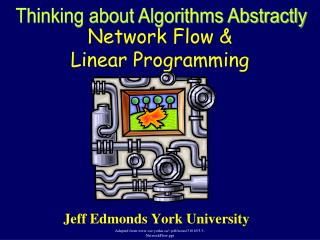DownloadDownload PresentationNetwork Flow & Linear Programming

# Network Flow & Linear Programming

Download Presentation## Network Flow & Linear Programming

- - - - - - - - - - - - - - - - - - - - - - - - - - - E N D - - - - - - - - - - - - - - - - - - - - - - - - - - -
##### Presentation Transcript

1. Thinking about Algorithms Abstractly Network Flow & Linear Programming Jeff Edmonds York University Adapted from www.cse.yorku.ca/~jeff/notes/3101/03.5-NetworkFlow.ppt

2. Network Flow • Instance: • A Network is a directed graph G • Edges represent pipes that carry flow • Each edge <u,v> has a maximum capacity c<u,v> • A source node s in which flow arrives • A sink node t out which flow leaves Goal: Max Flow Adapted from www.cse.yorku.ca/~jeff/notes/3101/03.5-NetworkFlow.ppt

3. Network Flow • Instance: • A Network is a directed graph G • Edges represent pipes that carry flow • Each edge <u,v> has a maximum capacity c<u,v> • A source node s in which flow arrives • A sink node t out which flow leaves Adapted from www.cse.yorku.ca/~jeff/notes/3101/03.5-NetworkFlow.ppt

4. Network Flow • Solution: • The amount of flow F<u,v> through each edge. • Flow can’t exceed capacity i.e. F<u,v> c<u,v>. • Unidirectional flow • F<u,v> 0 and F<v,u> = 0 • or • F<u,v> = 0 and F<v,u> 0 Some texts: F<u,v>= -F<v,u> Adapted from www.cse.yorku.ca/~jeff/notes/3101/03.5-NetworkFlow.ppt

5. Network Flow • Solution: • The amount of flow F<u,v> through each edge. • Flow F<u,v> can’t exceed capacity c<u,v>. • Unidirectional flow • No leaks, no extra flow. Adapted from www.cse.yorku.ca/~jeff/notes/3101/03.5-NetworkFlow.ppt

6. Network Flow Except for s and t. • Solution: • The amount of flow F<u,v> through each edge. • Flow F<u,v> cant exceed capacity c<u,v>. • Unidirectional flow • No leaks, no extra flow. • For each node v: flow in = flow out • u F<u,v> = w F<v,w> Adapted from www.cse.yorku.ca/~jeff/notes/3101/03.5-NetworkFlow.ppt

7. Network Flow • Value of Solution: • Flow from s into the network • minus flow from the network back into s. • rate(F) = u F<s,u> • = flow from network into t • minus flow back in. • = u F<u,t> - v F<t,v> - v F<v,s> What about flow back into s? Goal: Max Flow Adapted from www.cse.yorku.ca/~jeff/notes/3101/03.5-NetworkFlow.ppt

8. Network flow problem is a linear program Taken from www.infosun.fim.uni-passau.de/br/lehrstuhl/Kurse/Proseminar_ss01/Network_flow_problems.ppt

10. Network Flow A network with its edge capacities What is the maximum that can flow from s to t? Adapted from www.cse.yorku.ca/~jeff/notes/3101/03.5-NetworkFlow.ppt

11. Network Flow The max total rate of the flow is 1+2-0 = 3. flow/capacity = 2/5 A network with its edge capacities Can prove that total cannot be higher. Adapted from www.cse.yorku.ca/~jeff/notes/3101/03.5-NetworkFlow.ppt

12. Network Flow No more flow can be pushedalong the top path because theedge <b,c> is at capacity. Similarly, the edge <e,f>. No flow is pushed along the bottom path because this would decrease the total from s to t. Adapted from www.cse.yorku.ca/~jeff/notes/3101/03.5-NetworkFlow.ppt

13. Network Flow <U,V> is a minimum cut Its capacity is the sum of the capacities crossing the cut = 1+2 = 3. <i,j> is not included in because it is going in the wrong direction. Adapted from www.cse.yorku.ca/~jeff/notes/3101/03.5-NetworkFlow.ppt

14. Network Flow The edges crossing forward across the cut are at capacity those crossing backwards have zero flow. This is always true. Adapted from www.cse.yorku.ca/~jeff/notes/3101/03.5-NetworkFlow.ppt

15. Maxflow = Mincut The maximum flow is 1+2=3 The minimum cut is 1+2=3. These are always equal. Adapted from www.cse.yorku.ca/~jeff/notes/3101/03.5-NetworkFlow.ppt

16. An Application: Matching 3 matches Can we do better? 4 matches Who loves whom. Who should be matched with whomso as many as possible matchedand nobody matched twice? Adapted from www.cse.yorku.ca/~jeff/notes/3101/03.5-NetworkFlow.ppt

17. An Application: Matching s t 1 1 u v • c<s,u> = 1 • Total flow out of u = flow into u  1 • Boy u matched to at most one girl. • c<v,t> = 1 • Total flow into v = flow out of v  1 • Girl v matched to at most one boy. Adapted from www.cse.yorku.ca/~jeff/notes/3101/03.5-NetworkFlow.ppt

18. Min Cut s t • Instance: • A Network is a directed graph G • Special nodes s and t. • Edges represent pipes that carry flow • Each edge <u,v> has a maximum capacity c<u,v> • Partition into two regions so that the cut between the two is minimized Adapted from www.cse.yorku.ca/~jeff/notes/3101/03.5-NetworkFlow.ppt